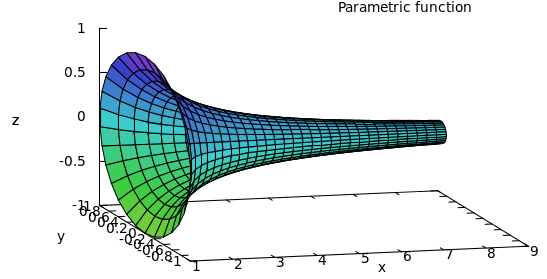13th September 2013

# Torricelli's Trumpet: Infinite Surface Area but Finite Volume

Just read on Torricelli's trumpet in Wikipedia. This states that there is a body having infinite surface but finite volume! That sounds contradictory at first.

Function in question is

$$y = 1 / x$$

from $$x=1$$ to $$a$$.Volume is

$$V = \pi \int_1^a \left({1\over x^2}\right)\,dx = \pi\left(1-{1\over a}\right)$$

Surface is

$$A = 2\pi \int_1^a {1\over x}\sqrt{1 + {1\over x^4}}\,dx \ge 2\pi \int_1^a {1\over x}\,dx = 2\pi\ln a$$

Above plot is from Maxima using:

plot3d( [x,1/x*sin(v),1/x*cos(v)], [x,1,8], [v,0,2*%pi] );